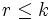# Congruence condition on number of subgroups of given prime power order

This article describes a congruence condition on an enumeration, or a count. It says that in a finite group and modulo prime number, the number of subgroups of given prime power order satisfies a congruence condition.
View other congruence conditions | View divisor relations

## Statement

### Version for a group of prime power order

Let$G$ be a group of prime power order$p^k$ and suppose$0 \le r \le k$. The following are true:

• The number of subgroups of$G$ of order$p^r$ is congruent to$1$ modulo$p$.
• The number of normal subgroups of$G$ of order$p^r$ is congruent to 1 mod$p$.
• The number of p-core-automorphism-invariant subgroups of$G$ of order$p^r$ is congruent to 1 mod$p$.

In particular, the collection of groups of order$p^r$ is a collection of groups satisfying a universal congruence condition.

### Version for a general finite group

Let$G$ be a finite group and$p^r$ be a prime power dividing the order of$G$. Then, the number of subgroups of$G$ of order$p^r$ is congruent to 1 mod$p$.

## Proof

### Equivalence between the multiple formulations

For a proof of the equivalence of the three formulations for a group of prime power order and the formulation for a general finite group, see collection of groups satisfying a universal congruence condition and equivalence of definitions of universal congruence condition.

### Proof for a group of prime power order in terms of all subgroups using Fact (1)

This proof uses the principle of mathematical induction in a nontrivial way (i.e., it would be hard to write the proof clearly without explicitly using induction).

This proof uses fact (1): congruence condition relating number of subgroups in maximal subgroups and number of subgroups in the whole group, combined with an induction on order.

Given: A group$G$ of order$p^k$.

To prove: For any$r \le k$, the number of subgroups of order$p^r$ is congruent to$1$ modulo$p$.

Proof: In this proof, we induct on$k$, i.e., we assume the statement is true inside groups of order$p^l, l \le k$.

Base case for induction: The case$k = 0$ is obvious.

Inductive step: If$r = k$, the number of subgroups is 1, so the statement is true. So we consider$r < k$.

For a subgroup$H$ of$G$, denote by$n(H)$ the number of subgroups of$H$ of order$p^r$.

Step no. Assertion/construction Facts used Given data/assumptions used Previous steps used Explanation
1$n(G) \equiv \sum_{M \operatorname{max} G} n(M) \pmod p$. Here,$M \operatorname{max} G$ means that$M$ is a maximal subgroup of$G$. Fact (1)$r < k$ [SHOW MORE]
2 For every$M \operatorname{max} G$,$n(M) \equiv 1 \pmod p$. inductive hypothesis
3 The number of maximal subgroups of$G$ is congruent to 1 mod$p$. Fact (2) Fact-direct.
4$n(G) \equiv 1 \pmod p$ Steps (1)-(3) [SHOW MORE]